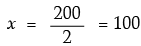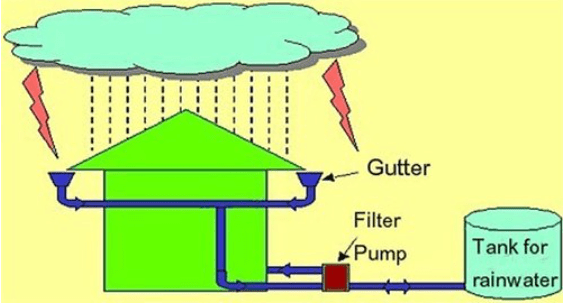Test: Linear Equations in Two Variables- Case Based Type Questions- 1

# Test: Linear Equations in Two Variables- Case Based Type Questions- 1 - EmSAT Achieve

Test Description

## 10 Questions MCQ Test Mathematics for EmSAT Achieve - Test: Linear Equations in Two Variables- Case Based Type Questions- 1

Test: Linear Equations in Two Variables- Case Based Type Questions- 1 for EmSAT Achieve 2023 is part of Mathematics for EmSAT Achieve preparation. The Test: Linear Equations in Two Variables- Case Based Type Questions- 1 questions and answers have been prepared according to the EmSAT Achieve exam syllabus.The Test: Linear Equations in Two Variables- Case Based Type Questions- 1 MCQs are made for EmSAT Achieve 2023 Exam. Find important definitions, questions, notes, meanings, examples, exercises, MCQs and online tests for Test: Linear Equations in Two Variables- Case Based Type Questions- 1 below.
Solutions of Test: Linear Equations in Two Variables- Case Based Type Questions- 1 questions in English are available as part of our Mathematics for EmSAT Achieve for EmSAT Achieve & Test: Linear Equations in Two Variables- Case Based Type Questions- 1 solutions in Hindi for Mathematics for EmSAT Achieve course. Download more important topics, notes, lectures and mock test series for EmSAT Achieve Exam by signing up for free. Attempt Test: Linear Equations in Two Variables- Case Based Type Questions- 1 | 10 questions in 20 minutes | Mock test for EmSAT Achieve preparation | Free important questions MCQ to study Mathematics for EmSAT Achieve for EmSAT Achieve Exam | Download free PDF with solutions
 1 Crore+ students have signed up on EduRev. Have you?
Test: Linear Equations in Two Variables- Case Based Type Questions- 1 - Question 1

### Prime Minister ’s National Relief Fund (also called PMNRF in short) is the fund raised to provide support for people affected by natural and manmade disasters. Natural disasters that are covered under this include flood, cyclone, earthquake etc. Man-made disasters that are included are major accidents, acid attacks, riots, etc.Two friends Sita and Gita, together contributed ₹ 200 towards Prime Minister’s Relief Fund. Answer the following :Q. How to represent the above situation in linear equations in two variables?

Detailed Solution for Test: Linear Equations in Two Variables- Case Based Type Questions- 1 - Question 1
x + y = 200

Here, x represents Sita’s contribution and y represents Gita’s contribution.

Test: Linear Equations in Two Variables- Case Based Type Questions- 1 - Question 2

### Prime Minister ’s National Relief Fund (also called PMNRF in short) is the fund raised to provide support for people affected by natural and manmade disasters. Natural disasters that are covered under this include flood, cyclone, earthquake etc. Man-made disasters that are included are major accidents, acid attacks, riots, etc.Two friends Sita and Gita, together contributed ₹ 200 towards Prime Minister’s Relief Fund. Answer the following :Q. If both contributed equally, then how much is contributed by each?

Detailed Solution for Test: Linear Equations in Two Variables- Case Based Type Questions- 1 - Question 2
If x = y then x + x = 200

2x = 200Thus, each contributed is ₹ 100.

Test: Linear Equations in Two Variables- Case Based Type Questions- 1 - Question 3

### Prime Minister ’s National Relief Fund (also called PMNRF in short) is the fund raised to provide support for people affected by natural and manmade disasters. Natural disasters that are covered under this include flood, cyclone, earthquake etc. Man-made disasters that are included are major accidents, acid attacks, riots, etc.Two friends Sita and Gita, together contributed ₹ 200 towards Prime Minister’s Relief Fund. Answer the following : Q. Which out of the following is not the linear equation in two variables ?

Detailed Solution for Test: Linear Equations in Two Variables- Case Based Type Questions- 1 - Question 3

x2 + x = 1

x2 + x = 1 is not linear as highest power is 2. Also, it is an equation in one variable.

Thus, it is not a linear equation in two variables.

Test: Linear Equations in Two Variables- Case Based Type Questions- 1 - Question 4

Prime Minister ’s National Relief Fund (also called PMNRF in short) is the fund raised to provide support for people affected by natural and manmade disasters. Natural disasters that are covered under this include flood, cyclone, earthquake etc. Man-made disasters that are included are major accidents, acid attacks, riots, etc.Two friends Sita and Gita, together contributed ₹ 200 towards Prime Minister’s Relief Fund. Answer the following :

Q. If Sita contributed ₹ 76, then how much was contributed by Gita ?

Detailed Solution for Test: Linear Equations in Two Variables- Case Based Type Questions- 1 - Question 4
If x = 76 then 76 + y = 200

y = 200 – 76

y = 124

Test: Linear Equations in Two Variables- Case Based Type Questions- 1 - Question 5

Prime Minister ’s National Relief Fund (also called PMNRF in short) is the fund raised to provide support for people affected by natural and manmade disasters. Natural disasters that are covered under this include flood, cyclone, earthquake etc. Man-made disasters that are included are major accidents, acid attacks, riots, etc.Two friends Sita and Gita, together contributed ₹ 200 towards Prime Minister’s Relief Fund. Answer the following :

Q. Which is the standard form of linear equation x = – 5 ?

Detailed Solution for Test: Linear Equations in Two Variables- Case Based Type Questions- 1 - Question 5
1.x + 0.y + 5 = 0

Since, x = 5

⇒ x + 5 = 0

Thus, standard form of x = – 5 is

1.x + 0.y + 5 = 0.

Test: Linear Equations in Two Variables- Case Based Type Questions- 1 - Question 6

Rainwater harvesting system is a technology that collects and stores rainwater for human use.

Anup decided to do rainwater harvesting. He collected rainwater in the underground tank at the rate of 30 cm3/sec.Q. What is the type of solution of the equation formed?

Detailed Solution for Test: Linear Equations in Two Variables- Case Based Type Questions- 1 - Question 6
Because for every value of x, there is a corresponding value of y and vice versa.
Test: Linear Equations in Two Variables- Case Based Type Questions- 1 - Question 7

Rainwater harvesting system is a technology that collects and stores rainwater for human use.

Anup decided to do rainwater harvesting. He collected rainwater in the underground tank at the rate of 30 cm3/sec.Q. How much water will be collected in 60 sec ?

Detailed Solution for Test: Linear Equations in Two Variables- Case Based Type Questions- 1 - Question 7
Since y = 30x

If x = 60, then, y = 30 × 60

= 1800

Required volume is 1800 cm3

Test: Linear Equations in Two Variables- Case Based Type Questions- 1 - Question 8

Rainwater harvesting system is a technology that collects and stores rainwater for human use.

Anup decided to do rainwater harvesting. He collected rainwater in the underground tank at the rate of 30 cm3/sec.Q. What will be the equation formed if volume of water collected in x seconds is taken as y cm3 ?

Detailed Solution for Test: Linear Equations in Two Variables- Case Based Type Questions- 1 - Question 8
Storage time of water = ‘x’ seconds

Amount of stored water = y cubic cm

Water stored per second = 30 cubic cm

Water stored in ‘x’ second = 30x

A.T.Q.

Amount of stored water = water in 'x' second

y = 30x

Test: Linear Equations in Two Variables- Case Based Type Questions- 1 - Question 9

Rainwater harvesting system is a technology that collects and stores rainwater for human use.

Anup decided to do rainwater harvesting. He collected rainwater in the underground tank at the rate of 30 cm3/sec.Q. Write the equation in standard form.

Detailed Solution for Test: Linear Equations in Two Variables- Case Based Type Questions- 1 - Question 9

Rainwater is collected in the  underground tank at the rate of 30 cm³ / sec

Water collected in 1 sec  = 30 cm³

Water collected in x secs = 30x  cm³

Water collected in x secs =  y cm³

=> y = 30x

a linear equation y = 30x

30x - y = 0 ,30x -y +0=0

is standard form

Test: Linear Equations in Two Variables- Case Based Type Questions- 1 - Question 10

Rainwater harvesting system is a technology that collects and stores rainwater for human use.

Anup decided to do rainwater harvesting. He collected rainwater in the underground tank at the rate of 30 cm3/sec.Q. How much time will it take to collect water in 900 cm3 ?

Detailed Solution for Test: Linear Equations in Two Variables- Case Based Type Questions- 1 - Question 10

Since y = 30x

If y = 900, then, 900 = 30x

X = 900 / 30 = 30

Required time is 30 sec.

## Mathematics for EmSAT Achieve

151 videos|237 docs|254 tests
Information about Test: Linear Equations in Two Variables- Case Based Type Questions- 1 Page
In this test you can find the Exam questions for Test: Linear Equations in Two Variables- Case Based Type Questions- 1 solved & explained in the simplest way possible. Besides giving Questions and answers for Test: Linear Equations in Two Variables- Case Based Type Questions- 1, EduRev gives you an ample number of Online tests for practice

## Mathematics for EmSAT Achieve

151 videos|237 docs|254 tests